Electron. J. Diff. Eqns., Vol. 2008(2008), No. 64, pp. 1-15.

### Matrix elements for sum of power-law potentials in quantum mechanic using generalized hypergeometric functions Qutaibeh D. Katatbeh, Ma'zoozeh E. Abu-Amra

Abstract:
In this paper we derive close form for the matrix elements for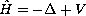, where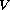is a pure power-law potential. We use trial functions of the form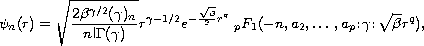for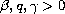to obtain the matrix elements for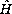. These formulas are then optimized with respect to variational parameters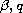and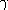to obtain accurate upper bounds for the given nonsolvable eigenvalue problem in quantum mechanics. Moreover, we write the matrix elements in terms of the generalized hypergeomtric functions. These results are generalization of those found earlier in , [8-16] for power-law potentials. Applications and comparisons with earlier work are presented.

Submitted February 19, 2008 Published April 28, 2008.
Math Subject Classifications: 34L15, 34L16, 81Q10, 35P15.
Key Words: Schrodinger equation; variational technique; eigenvalues; upper bounds; analytical computations.

Show me the PDF file (258 KB), TEX file, and other files for this article.Qutaibeh D. Katatbeh Department of Mathematics and Statistics, Faculty of Science and Arts Jordan University of Science and Technology Irbid 22110, Jordan email: qutaibeh@yahoo.com Ma'zoozeh E. Abu-Amra Department of Mathematics and Statistics, Faculty of Science and Arts Jordan University of Science and Technology Irbid 22110, Jordan